• matlab中find函数

万次阅读 多人点赞 2018-05-09 11:01:54
find函数：查询非零元素的位置和值用法1：B = find(A)，A是一个矩阵，查询非零元素的位置 如果A是一个行向量，则返回一个行向量，否则，返回一个列向量。 如果A全是零元素或者空数组，则返回一个空数组。&gt;&...

find函数：查询非零元素的位置和值

用法1：B = find(A)，A是一个矩阵，查询非零元素的位置

如果A是一个行向量，则返回一个行向量，否则，返回一个列向量。

如果A全是零元素或者空数组，则返回一个空数组。

>> A = [0 1 2 0 4 5]

A =
0     1     2     0     4     5

>> B = find(A)

B =
2     3     5     6

>> A = [0 1 2; 0 4 5]

A =
0     1     2
0     4     5

>> B = find(A)

B =
3
4
5
6
(位置的判定：在矩阵中，第一列开始，自上而下，依次为1，2，3...,然后再从第二列，第三列依次往后数)

用法2：B = find(A>2)，找到A中比2大的元素的位置

>> A = [0 1 2; 0 4 5]

A =
0     1     2
0     4     5

>> B = find(A>2)

B =
4
6

用法3：B = find(A，2)，找到A中最先出现的2个不为零的数的位置

>> A = [0 1 2; 0 4 5; 6 0 8]

A =
0     1     2
0     4     5
6     0     8

>> B = find(A, 2)

B =
3
4

用法4：B = find(A，2, 'first')，找到A中最先出现的2个不为零的数的位置

B = find(A，2, 'last')，找到A中最后出现的2个不为零的数的位置

>> A = [0 1 2; 0 4 5; 6 0 8]

A =
0     1     2
0     4     5
6     0     8

>> B = find(A, 2, 'first')

B =
3
4

>> C = find(A,2,'last')

C =
8
9

用法5：[r, c] = find(A)，找到A中非零元素所在的行和列，行存储在矩阵r中，列存储在矩阵c中

>> A = [0 1 2; 0 4 5; 6 0 8]

A =
0     1     2
0     4     5
6     0     8

>> [r, c] = find(A)

r =
3
1
2
1
2
3

c =
1
2
2
3
3
3

用法6：[r, c, v] = find(A)，找到A中非零元素所在的行和列，行存储在矩阵r中，列存储在矩阵c中，值存储在矩阵v中（需要知道值的用此用法，其他用法只能确定位置）

>> A = [0 1 2; 0 4 5; 6 0 8]

A =
0     1     2
0     4     5
6     0     8

>> [r, c, v] = find(A)

r =
3
1
2
1
2
3

c =
1
2
2
3
3
3

v =
6
1
4
2
5
8

展开全文matlab find
• matlab中find函数用法

万次阅读 多人点赞 2019-03-06 15:10:09
1.返回素有非零元素的位置 例如： 注：竖着数！！ 2.条件：find（A==1） 例如：返回的仍然是位置！ 3.返回前N个非零元素的位置,find(A,X...6.[a,b,v] = find(A)，找出A中非零元素所在的行和列，分别存储在a和b，...

1.返回素有非零元素的位置
例如：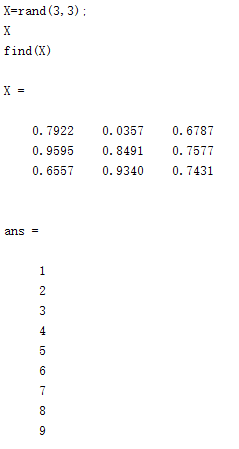注：竖着数！！
2.条件：find（A==1）
例如：返回的仍然是位置！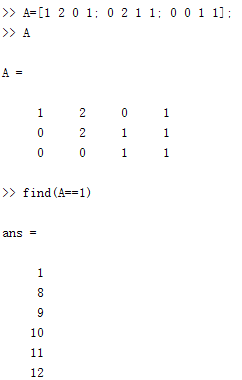3.返回前N个非零元素的位置,find(A,X)
例如：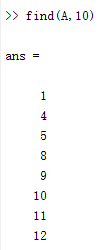4.返回最后一个非零值的位置find(A,1,‘last’)
例如：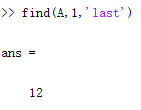5.返回最后一个非零值的行列位置或者A中非零元素位置
例如：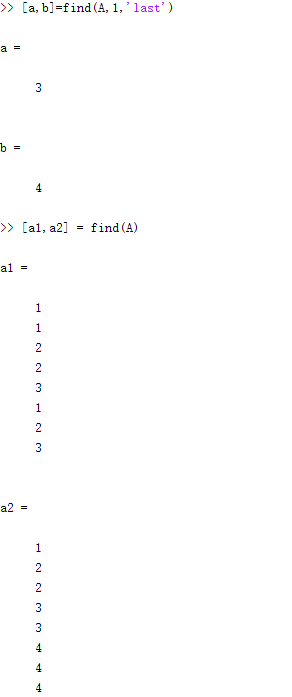6.[a,b,v] = find(A)，找出A中非零元素所在的行和列，分别存储在a和b中，并将结果放在v中.
例如：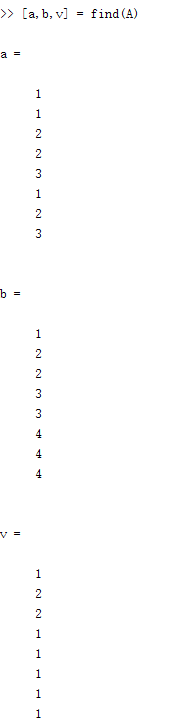展开全文matlab find函数
• Matlab中find函数的使用说明

千次阅读 2021-04-26 19:52:27
ind = find(X)找出矩阵X的所有非零元素，并将这些元素的线性索引值(linear indices：按列)返回到向量ind。如果X是一个行向量，则ind是一个行向量；否则，ind是一个列向量。如果X不含非零元素或是一个空矩阵，则...

find

找到非零元素的索引和值

语法：

1.  ind = find(X)

找出矩阵X中的所有非零元素，并将这些元素的线性索引值(linear indices：按列)返回到向量ind中。

如果X是一个行向量，则ind是一个行向量；否则，ind是一个列向量。

如果X不含非零元素或是一个空矩阵，则ind是一个空矩阵。

2.  ind = find(X, k) 或 3.  ind = find(X, k, 'first')

返回x中前k个非零元素的索引值。

k必须是一个正数，但是它可以是任何数字数值类型。

4.  ind = find(X, k, 'last')

返回后k个非零元素的索引值。

例如，一个5*7*3的矩阵X，有一个非零元素X(4,2,3)，find函数将返回row=4和col=16。也就是说，(第1页有7列)+(第2页有7列)+(第3页有2列)=16。

X=zeros(5,7,3);

X(4,2,3)=1;

[row,col]=find(X);

例子：

例1

X = [1 0 4 -3 0 0 0 8 6];

indices = find(X)

返回X中非零元素的线性索引值。

indices =

1     3     4     8     9

例2

你可以用一个逻辑表达方式定义X。

例如

find(X > 2)

返回X中大于2的元素的相对应的线性索引值。

ans =

3     8     9

例3

下面find指令

X = [3 2 0; -5 0 7; 0 0 1];

[r,c,v] = find(X)

返回X中非零元素行索引值的向量

r =

1

2

1

2

3

X中非零元素列索引值的向量

c =

1

1

2

3

3

包含X中非零元素的向量

v =

3

-5

2

7

1     (按列)

展开全文• matlab中find函数如何使用

千次阅读 2019-03-23 18:40:45
例子： a=[2 3 5 6 0 8 9] 1、 find(a),a是一个矩阵,查询非零元素的位置，如果X是一个行向量，则返回一个行向量，否则，...2、find(a,2),找出a最先出现的2个不为零的数,a是你所要找的矩阵,2参数是指数量；...

例子：

a=[2 3 5 6 0 8 9]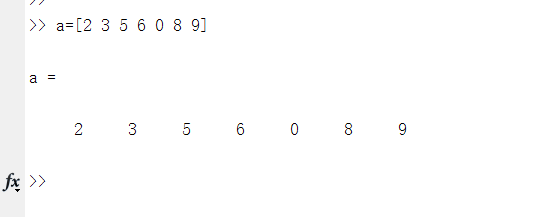1、 find(a),a是一个矩阵,查询非零元素的位置，如果X是一个行向量，则返回一个行向量，否则，返回一个列向量。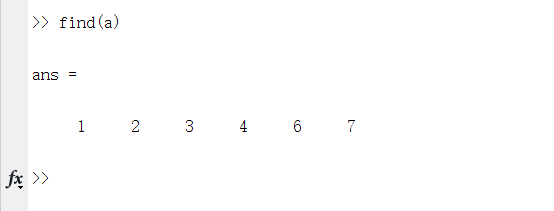如果X全是零元素或者是空数组，则返回一个空数组，例子如下所示,也可以用find(a>3)，这句的意思是输出在a中找到比较3大的元素的列数；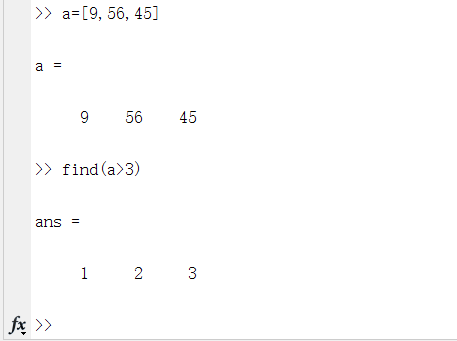2、find(a,2),找出a中最先出现的2个不为零的数,a是你所要找的矩阵,2参数是指数量；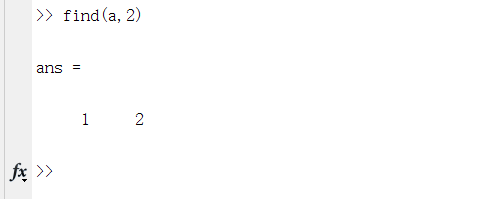必须为 ‘first’ 或 'last’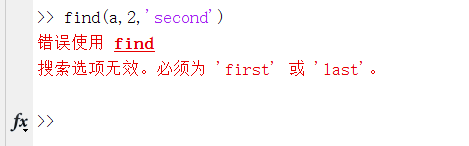3、find(a,2,‘first’)，用法和上例基本一样；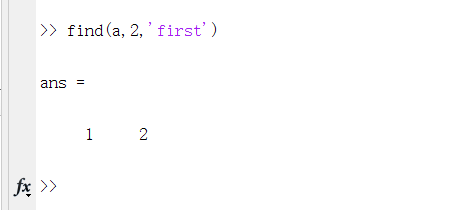4、find(a,2,‘last’) 这句的意思是从最后一个非零元素起，找2个不为零的元素，如下图所示；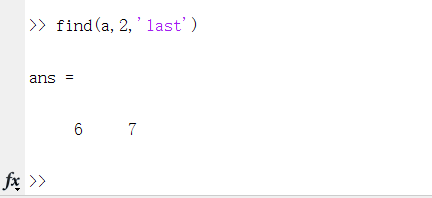5、[x,y]=find(a)，找出a矩阵中非零元素所在行和列，并存在x，y中；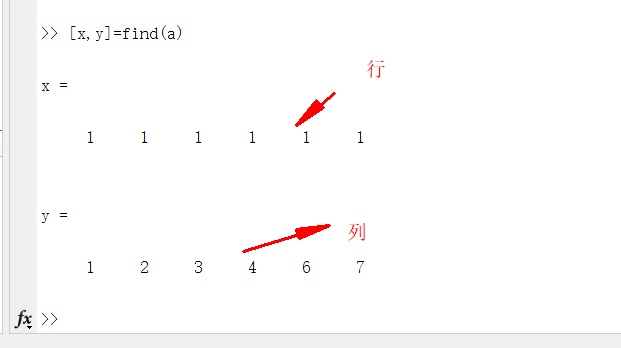找个明显的！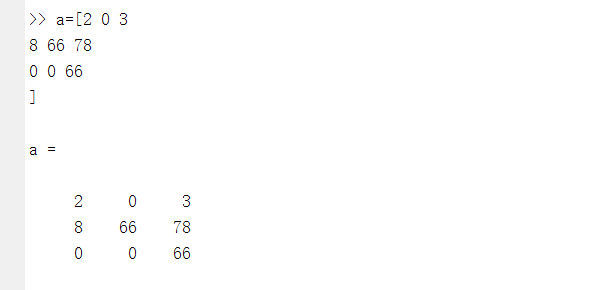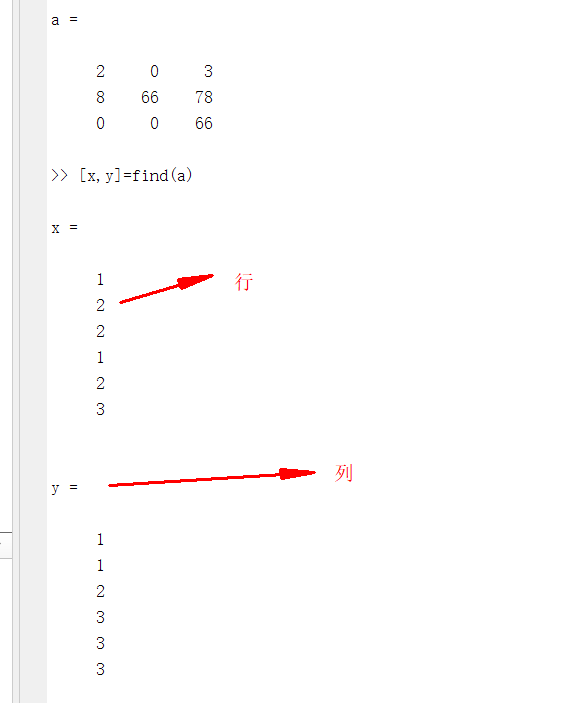6、[x,y,vv]=find(a)，找出矩阵中非零元素所在行和列,并存在x、y中，并将结果放在vv中，下图所示1表示行号，2表示列号，3表示矩阵a中的非零值；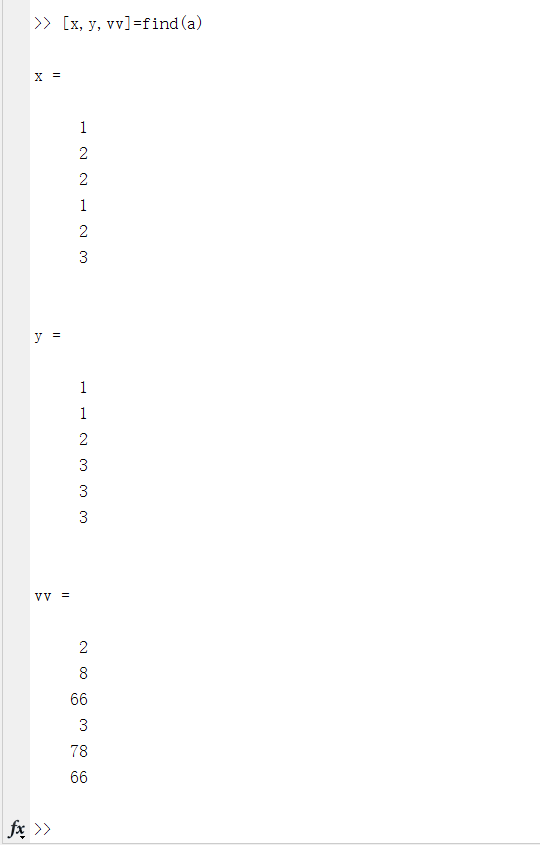展开全文• Matlab中find函数详解

千次阅读 2020-03-14 17:54:01
Matlab中find函数详解 在Matlab中通过“help find”指令可以看到find函数的作用是 “Find indices and values of nonzero elements”，即查找非零元素的索引(可以理解为位置)和值。常见用法如下： 一、假设数组a=[1 ...matlab
• MATLAB中find函数的用法

千次阅读 2018-12-14 13:45:34
MATLAB中find函数的用法 find函数：查找非零元素的索引和值 主要有下面5用法， k = find(X) X有三种情况： （1）：如果X为向量（行向量或者列向量），k为X中非零元素索引组成的向量。k的方向与X的方向相同。如果X...MATLAB
• MATLAB中Find函数使用方法 find函数 k = find(X)返回一个向量，其中包含数组X每个非零元素的线性索引。 如果X是一个向量，那么find返回一个与X方向相同的向量。 如果X是一个多维数组，那么find返回结果的线性索引...matlab
• MATLAB中find函数详解

万次阅读 多人点赞 2017-12-07 20:41:30
（转自http://blog.sina.com.cn/emily250886... 功能：  寻找非零元素的索引和值 语法： 1. ind = find(X) 2. ind = find(X, k) 3. ind = find(X, k, 'first') 4. ind = find(X, k, 'last') 5. [row,col]
• matlab中find函数可以返回指定元素的位置，但是有时候会发现矩阵明明存在一个数，但是返回位置却是空的，即找不到指定元素的位置。这时候需要考虑的是matlab的精度问题。如下图，表面上第一个元素是0.6678，...find matlab
• MATLABfind()函数

2015-11-14 09:13:51
• MATLAB中find函数用法

千次阅读 2015-09-12 21:03:43
find()函数是用来查询满足要求非零值在矩阵位置的函数，可以返回元素在其中的位置等，下面就通过实例介绍一下不同函数的用法。 返回向量中非零元素的位置 find(A) 我们以向量A=[0 1 2 3 4 5 6 7 8] 为例...find
• matlab中find函数的用法

万次阅读 多人点赞 2014-06-28 09:36:19
find   找到非零元素的索引和值     语法： 1. ind = find(X) 2. ind = find(X, k) 3. ind = find(X, k, 'first') 4. ind = find(X, k, 'last') 5. [row,col] = find(X, ...) 6. [row,col,v...matlab 函数用法
• MATLAB中 find() 函数的用法 matlab中find()函数用来找到矩阵中非零元素的相关信息。 根据返回参数的不同，有三种用法： 假设有矩阵M， (1) A = find(M); 该种用法直接返回M中非零元素按列存储的位置，A为一个列向量...matlab
• 该子函数为利用matlab中find函数改进，然后找出符合要求的目标的个数
• Matlabfind()函数 　当我第一次用matlab语言编写一个工程项目时，发现自己编写的脚本里循环特别多，导致编程效率很低，这让我特别苦恼。有一次导师让我阅读他编写的一个Matlab脚本，并按照新要求对其进行更改。...find
• MATLAB之find函数用法

千次阅读 2020-02-19 23:39:47
matlab find() 函数用法 I.用法一: b=find(a),a是一个矩阵,查询非零元素的位置，如果X是一个行向量，则返回一个行向量;否则，返回一个列向量。如果X全是零元素或者是空数组，则返回一个空数组,例子如下所示,也...matlab
• matlab中find（）函数用法

万次阅读 多人点赞 2020-10-14 19:59:41
注意：matlab中下标从1开始 举例： （1）向量 返回非零元素下标 find（vector） x=[1 2 3 0 0 6 7 8 9]; find(x) ans = 1 2 3 6 7 8 9 返回前k个非零元素的下标 find(vector,k) 或find（vector，k,‘first...matlab
• matlab中find函数的使用说明

万次阅读 2013-03-27 16:08:00
找到非零元素的索引和值     ...1. ind = find(X) ...2. ind = find(X, k) ...3. ind = find(X, k, 'first') ...4. ind = find(X, k, 'last') ...5. [row,col] = find(X, ...) ...6. [row,col,v] = find(X,
• a = [ 1 2 3 3 3]; 　如果a==3 　返回[ 0 0 1 1 1]; 　find(a==3) 　返回[3 4 5]，这才是想要的下标。
• 1. ind = find(X) 2. ind = find(X, k) 3. ind = find(X, k, 'first') 4. ind = find(X, k, 'last') 5. [row,col] = find(X, ...) 6. [row,col,v] = find(X, ...) 说明： 1. ind = find(X) 找出矩阵matlab 函数 find
• MATLAB中，很容易找到满足特定条件的值的索引：>> a = [1,2,3,1,2,3,1,2,3];>> find(a > 2) % find the indecies where this condition is true[3, 6, 9] % (MATLAB uses 1-based indexing)>>...
• http://jingyan.baidu.com/album/3c343ff71444890d3679635d.html?picindex=1matlab
• 使用matplotlib.mlab.find，不过在最近版本已经取消find函数 import matplotlib.mlab as mlab np_array = 一个2维数组 idx = mlab.find(np_array > 1) # idx为np_array，大于1的值的位置索引 方法2： 自...python matlab 矩阵
• MATLAB find()函数二维数组找最值 在python对应 np.where()函数，取最后一个结果就行！！ 简短截说，上代码 MATLAB源码： [max_Ix, max_Iy]=find(garyimg1==max(max(garyimg1))) max_Ix = max_Ix(1,1); max_Iy = ...
• 全栈工程师开发手册 （作者：栾鹏） matlab2c动态链接库下载 matlab库函数大全 matlab2c基础教程 matlab2c开发全解教程matlab2c调用方法...3、在cpp文件引入下面的代码#include "Matlab2c.h" #pramatlab c++ find
• Matlab中find函数用法

千次阅读 2014-08-05 08:57:02Matlab Find
• matlab中find函数简单用法

万次阅读 2010-05-22 10:48:00
a=[0 0 0 0 0 0 0 1 0 0 0 1 1 1 0 0 0 1 0 0 0 0 0 0 0] a=double(a); [i,j]=find(a>0.5);matlab
• 今天用find函数时，遇到了矩阵明明有某个值，find却总是找不到这个值。 A是一个矩阵，a是其中的一个值,idx=find(A==a)返回的idx是一个空的列表，即idx=[] 解决方法： 是精度问题。改成如下即可 idx=find(abs(A-a)&......

matlab中find函数matlab 订阅